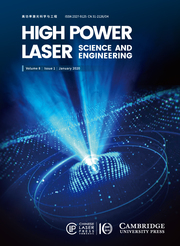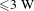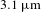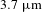Home
Hostname: page-component-65dc7cd545-sqtsj Total loading time: 0.285 Render date: 2021-07-23T15:00:17.948Z Has data issue: true Feature Flags: { "shouldUseShareProductTool": true, "shouldUseHypothesis": true, "isUnsiloEnabled": true, "metricsAbstractViews": false, "figures": true, "newCiteModal": false, "newCitedByModal": true, "newEcommerce": true, "newUsageEvents": true }High Power Laser Science and Engineering

# Highly efficient continuous-wave mid-infrared generation based on intracavity difference frequency mixing

Part of: HPL Letters

Published online by Cambridge University Press:  16 December 2019

Corresponding

## Abstract

We report on a new scheme for efficient continuous-wave (CW) mid-infrared generation using difference frequency generation (DFG) inside a periodically poled lithium niobate (PPLN)-based optical parametric oscillator (OPO). The pump sources were two CW fiber lasers fixed at 1018 nm and 1080 nm. One worked as the assisted laser to build parametric oscillation and generate an oscillating signal beam while the other worked at low power (${\leqslant}3~\text{W}$ ) to induce DFG between it and the signal beam. The PPLN temperature was appropriately adjusted to enable OPO and DFG to synchronously meet phase-matching conditions. Finally, both low-power 1018 nm and 1080 nm pump beams were successfully converted to$3.1~\unicode[STIX]{x03BC}\text{m}$ and$3.7~\unicode[STIX]{x03BC}\text{m}$ idler beams, respectively. The conversion efficiencies of the 1018 nm and 1080 nm pumped DFG reached 20% and 15%, respectively, while their slope efficiencies reached 19.6% and 15%. All these data were comparable to the OPOs pumped by themselves and never realized before in traditional CW DFG schemes. The results reveal that high-efficiency frequency down-conversion can be achieved with a low-power near-infrared pump source.

## Keywords

Type
Letter
Information
Creative CommonsThis is an Open Access article, distributed under the terms of the Creative Commons Attribution licence (http://creativecommons.org/licenses/by/4.0/), which permits unrestricted re-use, distribution, and reproduction in any medium, provided the original work is properly cited.

Because they cover the important transparent window of the atmosphere and nearly all fundamental rovibrational absorption bands of molecules, mid-infrared lasers have been widely used in the areas of environmental monitoring, medical diagnosis and counter-measurement[Reference Petrov, Goldberg, Burns, Curl and Tittel1Reference Bekman, van den Heuvel, van Putten and Schleijpen3]. The optical parametric oscillator (OPO) is an effective method to obtain high-power, continuous-wave (CW) mid-infrared coherent lasers, and has been extensively investigated[Reference Wu, Wang, Song, Ye, Xu, Li and Zhou4Reference Klein, Gross, Boller, Auerbach, Wessels and Fallnich7]. However, it usually has a high threshold, especially for CW pump sources. Based on this, difference frequency generation (DFG) is an alternate approach that may provide some advantages. The overall OPO system is much simpler than the dual-wavelength (DW) pump scheme. However, it is seriously limited by many pump sources which have difficulty in providing amplifying power. The DFG process is nonresonant, and avoids the need for a resonant cavity, significantly low reflectances on the crystal surfaces, complicated coatings on mirrors, or critical cavity alignment to initiate. By controlling the pump wavelength, crystal temperature or grating period, wide tunability of DFG power in the wavelength range$3.1{-}4~\unicode[STIX]{x03BC}\text{m}$ has also been demonstrated[Reference Mao, Jiang, Li, Chang and Liu8Reference Goldberg, Burns and McElhanon10]. Periodically poled lithium niobate (PPLN) is an attractive nonlinear crystal for the DFG process to generate mid-infrared lasers due to its low absorption at the interacting wavelengths, large interaction length (50 mm), relatively high value of the second-order nonlinear optical coefficient and absence of beam walk-off. In 1995, Goldberg et al. first demonstrated$3.0{-}4.1~\unicode[STIX]{x03BC}\text{m}$ tunability and a 0.5 mW CW idler output power at$3.02~\unicode[STIX]{x03BC}\text{m}$ using DFG in a bulk PPLN by mixing 420 mW at$0.787~\unicode[STIX]{x03BC}\text{m}$ and 4 W at$1.064~\unicode[STIX]{x03BC}\text{m}$ [Reference Goldberg, Burns and McElhanon10]. In 2014, Guha et al. successfully achieved 3.55 W CW idler output at$3.4~\unicode[STIX]{x03BC}\text{m}$ by single-pass difference frequency mixing of 43.3 W and 31 W at$1.064~\unicode[STIX]{x03BC}\text{m}$ and$1.55~\unicode[STIX]{x03BC}\text{m}$ , respectively, incident on a PPLN crystal, which was the highest CW mid-infrared power generated by the DFG process to date[Reference Guha, Barnes and Gonzalez11]. Recently, Zhao et al. generated a CW mid-infrared single-frequency DFG source tunable from 3.1 to$3.6~\unicode[STIX]{x03BC}\text{m}$ , using a PPLN crystal. The maximum idler output power reached${\sim}62.4~\text{mW}$ with incident pump and signal laser powers of 23.2 and 4.31 W, respectively, which was the highest reported power for a wavelength-tunable single-frequency CW DFG source[Reference Zhao, Jia, Feng and Nission12]. In 2018, we demonstrated a DW mid-infrared OPO which was pumped by two independent fiber lasers fixed at 1018 and 1080 nm, and realized phase-matched signal beam coupling by tuning the grating period of the PPLN. However, limited by the crystal and tuning method, the slope and conversion efficiencies of both the OPO processes were not enhanced.

Although mid-infrared CW DFG sources have been extensively researched, their pump-to-idler conversion efficiency was relatively low compared with OPOs, which resulted from the low single-pass parametric gain. Furthermore, a signal laser around$1.5~\unicode[STIX]{x03BC}\text{m}$ with a power of tens of watts or higher is needed if the power of the pump laser around$1~\unicode[STIX]{x03BC}\text{m}$ is relatively low. However, an obvious fact is that a high-power signal laser exists inside the cavity of the OPO pumped by the$1~\unicode[STIX]{x03BC}\text{m}$ fiber laser, and its wavelength can be easily tuned by changing the pump wavelength, crystal temperature or grating period. It can be speculated that the DFG efficiency and output power can be greatly enhanced if it takes place inside the OPO cavity and the phase-matching conditions are satisfied. To prove this assumption, in this letter, we report on the efficient frequency down-conversion of CW 1018 and 1080 nm fiber lasers using intracavity DFG in a PPLN-based OPO. The two pump sources were connected in parallel for independent tuning. One fiber laser worked at high power and served as the assisted laser to build parametric oscillation and generate the signal beam, while the other worked at low power and participated in the DFG between it and the signal beam. The PPLN temperature was appropriately tuned to enable the OPO and DFG to simultaneously satisfy phase matching. Ultimately, the low-power 1018 nm and 1080 nm pump beams were converted to mid-infrared radiation fixed at$3.1~\unicode[STIX]{x03BC}\text{m}$ and$3.7~\unicode[STIX]{x03BC}\text{m}$ , respectively. The conversion efficiencies of 1018 nm and 1080 nm pumped DFG reached 20% and 15%, respectively, and their slope efficiencies were 19.6% and 15%. All these data were just a little lower than that of the OPOs pumped by themselves. The efficiency could be further increased by enhancing the pump power of the assisted fiber laser or controlling the crystal temperature more precisely. This is, for the first time to our knowledge, the highest result for CW single-pass DFG schemes and a totally new scheme to realize high-efficiency CW mid-infrared generation using a low-power CW pump beam. It reveals the great prospects for applications in frequency down-conversion of specialized laser sources such as single-frequency, ultrafast laser sources, which are of great use in the mid-infrared range.

The experimental setup is shown in Figure 1. Two CW linearly polarized fiber lasers were adopted as the pump sources, whose central wavelengths were 1018 nm and 1080 nm, respectively. The maximum 1018 nm and 1080 nm output power reached 40 W and 28 W, respectively, with a full-width at half-maximum (FWHM) of 0.22 nm and 0.5 nm. They were combined in parallel by a$1018/1080~\text{nm}$ polarization-maintaining (PM) wavelength division multiplexer (WDM) whose maximum power-handling capacity was 50 W. As depicted in Figure 1, two ports of the WDM, port 1 and port 2, were connected with two fiber lasers and the DW pump beam was output from port 3, while about 1% of the total power was output from port 4 for spectral measurements to monitor the working status of the two fiber lasers. Port 3 was terminated with a collimator that produced a 1.6-mm-diameter collimated beam. After reflection from two gold mirrors, the pump beam was focused into the OPO cavity with a beam radius of$200~\unicode[STIX]{x03BC}\text{m}$ at the center of the crystal by a 15-cm-focal-length, anti-reflection (AR) coated focusing lens. The OPO cavity had a typical linear structure, consisting of two$\text{CaF}_{2}$ concave mirrors, M1 and M2. The radii of curvature of both M1 and M2 were 100 mm and the total cavity length was designed as 120 mm. Both M1 and M2 had an anti-reflection coating ($R<5\%$ ) for the pump over the range$1{-}1.2~\unicode[STIX]{x03BC}\text{m}$ and the idler over the range$3{-}4~\unicode[STIX]{x03BC}\text{m}$ . M1 had a high-reflection coating ($R>99\%$ ) for the signal over the range$1.4{-}1.7~\unicode[STIX]{x03BC}\text{m}$ and M2 was the output coupling mirror, having about 5% transmittance for the signal, thus ensuring singly resonant oscillation operation for the signal beam. The nonlinear crystal was a bulk 5% (mole fraction) MgO-doped PPLN with dimensions of$50~\text{mm}\times 10~\text{mm}\times 1~\text{mm}$ , which had a grating period of$29.84~\unicode[STIX]{x03BC}\text{m}$ and was placed between M1 and M2. Both two end faces of the PPLN were carefully polished and coated with anti-reflection coatings for all three wavelength bands. An oven was placed under the cavity to provide temperature control. The output beam was collimated by an uncoated$\text{CaF}_{2}$ lens. Dichroic beam splitters, M3, M4 and M5, separated the residual pump, signal and two idler beams.Figure 1. Schematic diagram of efficient mid-infrared generation based on intracavity DFG.Figure 2. (a) Simulated signal wavelength versus grating period at different crystal temperatures. (b) Simulated tuning curves for$55\,^{\circ }\text{C}$ with$1018~\text{nm}/1080~\text{nm}$ pumping.

To make effective use of the high-power intracavity signal beam for realizing efficient DFG, the DFG process needs to satisfy phase-matching conditions. Using the often-cited$\text{LiNbO}_{3}$ Sellmeier equations from Edwards and Lawrence[Reference Edwards and Lawrence13] and a more recent equation from Jundt[Reference Jundt14], Figure 2(a) depicts the simulated signal wavelength versus the grating period at different temperatures when the OPO was simultaneously pumped by 1018 and 1080 nm wavelengths. In the figure, every pair of curves with the same color represent 1018 and 1080 nm tuning curves at the same temperature and the dotted deep-blue line represents the grating period, which equals$29.84~\unicode[STIX]{x03BC}\text{m}$ . Based on the experimental conditions, the temperature in the simulation was set from 30 to$70\,^{\circ }\text{C}$ in steps of$5\,^{\circ }\text{C}$ . To illustrate the meaning more clearly, the ordinate value was just set from 1510 to 1513 nm, part of the signal wavelength range. The figure reveals that the 1018 nm tuning curve always has an intersection with the 1080 nm tuning curve in the range 1510 to 1513 nm when the grating period changes from 29.76 to$29.94~\unicode[STIX]{x03BC}\text{m}$ , regardless of the temperature change. It indicates that if the grating period is exactly located at the intersection, the two pump beams can share the same signal beam and ensure phase matching simultaneously, regardless of whether there are two OPOs or an OPO and DFG. It is obvious that the most appropriate temperature for a period of$29.84~\unicode[STIX]{x03BC}\text{m}$ to realize this purpose is$55\,^{\circ }\text{C}$ . To illustrate the theory more exactly, the complete tuning curves corresponding to$1018~\text{nm}/1080~\text{nm}$ pumping when the temperature is set as$55\,^{\circ }\text{C}$ were simulated and are shown in Figure 2(b). The figure indicates that when the grating period is$29.84~\unicode[STIX]{x03BC}\text{m}$ , the phase-matched signal wavelength for 1018 nm and 1080 nm pump beams was 1511.7 nm and 1511.4 nm, respectively. The 0.3 nm wavelength interval was much smaller than that of the DFG gain bandwidth, which was about 3 nm[Reference Samanta, Aadhi and Ebrahim-Zadeh15]. It means that once the high-power$1018/1080~\text{nm}$ pump beam builds parametric oscillation, the low-power$1080/1018~\text{nm}$ pump beam is able to realize efficient frequency down-conversion based on the DFG process between it and the intracavity signal beam. Both Figures 3(a) and 3(b) indicate that the crystal temperature should be controlled to around$55\,^{\circ }\text{C}$ to ensure the DFG process satisfies phase-matching conditions for efficient frequency down-conversion of the$1018/1080~\text{nm}$ pump beam.Figure 3. Measured idler and signal spectra under different pumping conditions when the PPLN temperature was controlled at$55\,^{\circ }\text{C}$ .

According to the simulation results, the PPLN was heated to$55\,^{\circ }\text{C}$ . The power of one fiber laser was limited to be no higher than 3 W and the other worked at the maximum to avoid two OPOs, but whilst realizing one OPO and one DFG. The signal and idler spectra were measured under$3/28~\text{W}$ of$1018/1080~\text{nm}$ pumping and$40/2.4~\text{W}$ of$1018/1080~\text{nm}$ pumping, respectively, and are shown in Figure 3. In the two cases, the high-power pump beam was used to generate the intracavity signal beam while the low-power pump beam was used to induce DFG. As can be seen from the figure, just one signal beam was detected in the two cases. Its central wavelength was 1513 nm under strong 1080 nm pumping and changed to 1512 nm under strong 1018 nm pumping. The FWHM values of the 1513 nm and 1512 nm signal waves were 85 pm and 90 pm, respectively. This phenomenon indicated that just one parametric oscillation existed in the cavity. In the two cases, the idler spectra always had two peaks, which were located at 3112 nm and 3778 nm under strong 1080 nm pumping, changing to 3116 nm and 3784 nm under strong 1018 nm pumping. The$3778/3784~\text{nm}$ and$3112/3116~\text{nm}$ idler beams are denoted for short as 3.7 and$3.1~\unicode[STIX]{x03BC}\text{m}$ idler beams in the following content. It was found that under strong 1080 nm pumping, the$3.7~\unicode[STIX]{x03BC}\text{m}$ idler power was higher than that under strong 1018 nm pumping. Greater thermal effects were produced because the absorption coefficient of the PPLN increased with the laser wavelength in the mid-infrared range[Reference Vainio, Peltola, Persijn, Harren and Haolnen16], and led to a longer signal wavelength and a shorter idler wavelength under strong 1080 nm pumping. Apparently, in the two cases, the low-power pump beam was not able to build independent parametric oscillation due to its low power. Thus, it was easy to draw the conclusion that under strong 1080 nm pumping, the$3.7~\unicode[STIX]{x03BC}\text{m}$ idler beam was generated by the 1080 nm pump beam based on parametric oscillation while the$3.1~\unicode[STIX]{x03BC}\text{m}$ idler beam was generated by the 1018 nm pump beam based on DFG between it and the 1513 nm signal beam. The conditions under strong 1018 nm pumping were just the reverse. Benefitting from the high-power intracavity signal beam and phase matching, the low-power pump beam was able to achieve frequency down-conversion at every power level. Here we do not present all measured spectra under different pump powers. The experimental results basically agreed with the simulation in Figure 2(b). The generated signal wavelength was a little longer than the simulation results. It was found that the thermal effect in the PPLN crystal was the main reason for this phenomenon. The PPLN crystal absorbed part of the laser energy when the OPO operated and led to a temperature rise that caused the signal wavelength to be a little longer than the theoretical value. In addition, the whole OPO system used in this experiment has been integrated and modulated, just as described in Ref. [Reference Shang, Xu, Wang, Li, Zhou and Xu17]. The oven could only be placed under the whole OPO module, resulting in rough control of the crystal temperature, and the control accuracy of the oven was just$0.5\,^{\circ }\text{C}$ , which was not precise enough to achieve excellent phase matching.Figure 4. Measured 3.1 and$3.7~\unicode[STIX]{x03BC}\text{m}$ idler power, conversion efficiency of 1018 and 1080 nm pump waves (a) versus the 1018 nm pump power under strong 1080 nm pumping and (b) versus the 1080 nm pump power under strong 1018 nm pumping. Inset in (a): power scaling of 1018 nm pumped OPO. Inset in (b): power scaling of 1080 nm pumped OPO.

The 3.1 and$3.7~\unicode[STIX]{x03BC}\text{m}$ idler power as well as the conversion efficiency of the 1018 nm and 1080 nm pump waves as a function of 1018 nm pump power was measured under strong 1080 nm pumping and is shown in Figure 4(a). The maximum$3.1~\unicode[STIX]{x03BC}\text{m}$ idler power reached 594 mW when the 1018 nm pump power was 3 W, indicating a 19.6% pump-to-idler slope efficiency, and the growth trend was very steady. This is, to the best of our knowledge, the highest result for CW DFG schemes. The conversion efficiency of the 1018 nm pumped DFG ranged from 20% to 22%, which was also sufficiently high compared with traditional single-pass DFG. During the DFG process, the$3.7~\unicode[STIX]{x03BC}\text{m}$ idler power and conversion efficiency decreased from 3.57 to 3.33 W and from 12.7% to 11.9%, respectively. It indicates that the 1018 nm pumped DFG process did not seriously affect the 1080 nm pumped OPO process, ensuring an adequate intracavity signal power and realization of efficient DFG. For comparison, the OPO was also pumped by a single 1018 nm fiber laser and the idler power versus pump power is shown in the inset of Figure 4(a). It reveals that the maximum$3.1~\unicode[STIX]{x03BC}\text{m}$ idler power reached 9.4 W under 40 W pump power. The slope efficiency was 24% and the conversion efficiency ranged from 23% to 29%. Both of them were better than the 1018 nm pumped DFG. There were two main reasons. One was that the phase mismatch in the 1018 nm pumped DFG was certainly greater than in the 1018 nm pumped OPO, which would lead to lower efficiency. The other reason was that the signal power inside the 1080 nm pumped OPO was certainly much lower than the 1018 nm pumped OPO, which would also result in lower efficiency DFG. Figure 4(b) depicts characteristics of the 1080 nm pumped DFG under strong 1018 nm pumping. It can be seen that the maximum$3.7~\unicode[STIX]{x03BC}\text{m}$ idler power reached 0.36 W when the 1080 nm pump power was 2.4 W and the slope efficiency was 15%. The conversion efficiency of DFG was basically fixed at 15%. During the whole DFG process, the$3.1~\unicode[STIX]{x03BC}\text{m}$ idler power and conversion efficiency decreased slightly from 9.3 to 9 W and from 23.5% to 22.5%, and ensured efficient realization of 1080 nm pumped DFG. The inset in Figure 4(b) reveals that the 1080 nm pumped OPO had a maximum idler power of 3.43 W under 28 W pump power and the slope efficiency was 17.3%. The conversion efficiency ranged from 9.25% to 12.25%. It can be seen that although the phase mismatch in the 1080 nm pumped DFG was greater than in the 1080 nm pumped OPO, the efficiency of DFG was almost comparable to that of OPO, which benefitted from the high signal power inside the 1018 nm pumped OPO. Furthermore, Figure 4 indicates that the thresholds of the 1018 nm and 1080 nm pumped OPO were 5.1 W and 10 W, respectively, and verified that both the 3 W 1018 nm pump beam and the 2.4 W 1080 nm pump beam were not able to build independent OPO. It can be speculated that the performance of DFG could be improved by using a higher power assisted laser and more precise temperature control. Some specialized near-infrared fiber sources such as single-frequency lasers, high-speed swept lasers and ultra-short pulsed lasers would be of great use if they were converted to the mid-infrared range. However, as they are limited by fiber nonlinear effects, it is very difficult to amplify them to high enough power to pump the OPO independently. The experimental results shown in Figure 4 offer effective assistance to realize frequency down-conversion of these low-power specialized fiber lasers using phase-matched intracavity difference frequency mixing inside high-power OPOs.

In conclusion, we have proposed a new scheme for realizing efficient mid-infrared generation using phase-matched intracavity DFG in a PPLN-based OPO. Two fiber lasers fixed at 1018 and 1080 nm were adopted as pump sources. One fiber laser worked as the assisted laser at maximum power to build parametric oscillation and generate an intracavity signal beam, while the other one worked at low power to participate in DFG between it and the signal beam. To realize efficient DFG, the PPLN temperature was appropriately tuned to$55\,^{\circ }\text{C}$ to enable OPO and DFG processes to synchronously satisfy phase-matching conditions. Finally, both low-power 1018 nm and 1080 nm pump beams were successfully converted to the mid-infrared range, which was fixed at$3.1~\unicode[STIX]{x03BC}\text{m}$ and$3.7~\unicode[STIX]{x03BC}\text{m}$ , respectively. The slope and conversion efficiencies of the 1018 nm pumped DFG reached 19.6% and 20%, while that of the 1080 nm pumped OPO reached 15% and 15%. This is, to the best of our knowledge, the highest result for CW single-pass DFG schemes. Although the DFG results were slightly inferior to the OPO pumped by themselves, the efficiency is expected to be improved by enhancing the assisted pump power or achieving more precise temperature control. This new scheme reveals the strong potential for frequency down-conversion of some specialized near-infrared laser sources that would be of great use if converted to the mid-infrared range.

## Acknowledgements

This work was supported by the Open Research Fund of State Key Laboratory of Pulsed Power Laser Technology, China (No. SKL2017KF04). We thank Nanjing University, Zhejiang University and Fujian Institute of Research on the Structure of Matter for their offer of nonlinear crystals.

## References

Petrov, K. P. Goldberg, L. Burns, W. K. Curl, R. F. and Tittel, F. K. Opt. Lett. 21, 86 (1996).CrossRefGoogle Scholar
Willer, U. Saraji, M. Khorsandi, A. Geiser, P. and Schade, W. Opt. Lasers Eng. 44, 699 (2006).CrossRefGoogle Scholar
Bekman, H. H. P. T. van den Heuvel, J. C. van Putten, F. J. M. and Schleijpen, H. M. A. Proc. SPIE 5615, 27 (2004).CrossRefGoogle Scholar
Wu, H. Wang, P. Song, J. Ye, J. Xu, J. Li, X. and Zhou, P. Opt. Express 26, 6446 (2018).CrossRefGoogle Scholar
Aadhi, A. Sharma, V. Singh, R. P. and Samanta, G. K. Opt. Lett. 42, 3674 (2017).CrossRefGoogle Scholar
Ly, A. Siour, C. and Bretenaker, F. Opt. Express 25, 9049 (2017).CrossRefGoogle Scholar
Klein, M. E. Gross, P. Boller, K.-J. Auerbach, M. Wessels, P. and Fallnich, C. Opt. Lett. 28, 920 (2003).CrossRefGoogle Scholar
Mao, Q. H. Jiang, J. Li, X. Q. Chang, J. H. and Liu, W. Q. Laser Phys. Lett. 6, 647 (2009).CrossRefGoogle Scholar
Krzempek, K. Sobon, G. Sotor, J. Dudzik, G. and Abramski, K. M. Laser Phys. Lett. 11, 105103 (2014).CrossRefGoogle Scholar
Goldberg, L. Burns, W. K. and McElhanon, R. W. Opt. Lett. 20, 1280 (1995).CrossRefGoogle Scholar
Guha, S. Barnes, J. O. and Gonzalez, L. P. Opt. Lett. 39, 5018 (2014).CrossRefGoogle Scholar
Zhao, J. Jia, F. Feng, Y. and Nission, J. IEEE J. Sel. Top. Quantum Electron. 24, 3100208 (2018).Google Scholar
Edwards, G. J. and Lawrence, M. Opt. Quant. Electron. 16, 373 (1984).CrossRefGoogle Scholar
Jundt, D. H. Opt. Lett. 22, 1553 (1997).CrossRefGoogle Scholar
Vainio, M. Peltola, J. Persijn, S. Harren, F. J. M. and Haolnen, L. Appl. Phys. B 94, 411 (2009).CrossRefGoogle Scholar
Shang, Y. Xu, J. Wang, P. Li, X. Zhou, P. and Xu, X. Opt. Express 24, 21684 (2016).CrossRefGoogle ScholarYou have AccessOpen access
8
Cited by

# Send article to Kindle

Note you can select to send to either the @free.kindle.com or @kindle.com variations. ‘@free.kindle.com’ emails are free but can only be sent to your device when it is connected to wi-fi. ‘@kindle.com’ emails can be delivered even when you are not connected to wi-fi, but note that service fees apply.

Find out more about the Kindle Personal Document Service.

Highly efficient continuous-wave mid-infrared generation based on intracavity difference frequency mixing
Available formats
×

# Send article to Dropbox

To send this article to your Dropbox account, please select one or more formats and confirm that you agree to abide by our usage policies. If this is the first time you use this feature, you will be asked to authorise Cambridge Core to connect with your <service> account. Find out more about sending content to Dropbox.

Highly efficient continuous-wave mid-infrared generation based on intracavity difference frequency mixing
Available formats
×

# Send article to Google Drive

To send this article to your Google Drive account, please select one or more formats and confirm that you agree to abide by our usage policies. If this is the first time you use this feature, you will be asked to authorise Cambridge Core to connect with your <service> account. Find out more about sending content to Google Drive.

Highly efficient continuous-wave mid-infrared generation based on intracavity difference frequency mixing
Available formats
×
×